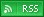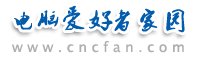电脑爱好者，提供IT资讯信息及各类编程知识文章介绍，欢迎大家来本站学习电脑知识。 最近更新 | 联系我们站内搜索：

# PHP4和PHP5对比分析：构造函数和析构函数

class foo {
var \$x;
function __construct(\$x) {
\$this->x = \$x;
}
function display() {
print(\$this->x);
}
function __destruct() {
print("bye bye");
}
}
\$o1 = new foo(4);
\$o1->display();
?>

class foo {
var \$x;
function setX(\$x) {
\$this->x = \$x;
}
function getX() {
return \$this->x;
}
}
\$o1 = new foo;
\$o1->setX(4);
\$o2 = \$o1;
\$o1->setX(5);
if(\$o1->getX() == \$o2->getX()) print("Oh my god!");
?>

class foo {
var \$x;
function setX(\$x) {
\$this->x = \$x;
}
function getX() {
return \$this->x;
}
}
\$o1 = new foo;
\$o1->setX(4);
\$o2 = \$o1->__clone();
\$o1->setX(5); if(\$o1->getX() != \$o2->getX()) print（"Copies are independant"）;
?>

 PHP热门文章排行 网站赞助商